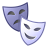# New to Qlik Sense

If you’re new to Qlik Sense, start with this Discussion Board and get up-to-speed quickly.

Announcements
QlikWorld 2023, a live, in-person thrill ride. Save \$300 before February 6: REGISTER NOW!
cancel
Showing results for
Did you mean:Creator II

## Problem with 80/20 split pie chart

Hi Qlikers,

I want to create 80/20 split pie chart for sales.

In the dimension i am using - ValueList(80, 20)

In the measure i am using -

Pick(

ValueList(80, 20),

count(Aggr(If(Rangesum(Above(Sum([Sales)/Sum(TOTAL [Sales]),0,RowNo()))<0.8,[Region]),([Region],(=Sum([Sales]),Desc))))

,count(Aggr(If(Rangesum(Above(Sum([Sales])/Sum(TOTAL [Sales]),0,RowNo()))>0.8,[Region]),([Region],(=Sum([Sales]),Desc))))

)

Now, the problem is that it shows the correct value for 80 but it doesn't show any value for 20. How do i tweak the expression to get the the result for 20 as well?

Any help would be greatly appreciated.

Thank you!Contributor III

=Pick(Match(ValueList(80,20),80,20),

count(Aggr(If(Rangesum(Above(Sum([Sales])/Sum(TOTAL [Sales]),0,RowNo()))<0.8,[Region]),[Region]))

,count(Aggr(If(Rangesum(Above(Sum([Sales])/Sum(TOTAL [Sales]),0,RowNo()))>=0.8,[Region]),[Region])))

You had missed the closing square bracket around the 'Sales' field. See the bracket in bold above. There were additional dimensions in your aggr() function which I'm not sure were needed. You won't need the '=' in your sum([Sales]) expression inside the aggr() if you do put it back in.

Also, I've added the criteria '>= 0.8' because anything on exactly 0.8 will be missing if you just use '<>0.8' between the calculations.

Hopefully this gives you something to work with.

SamTags
Community Browser